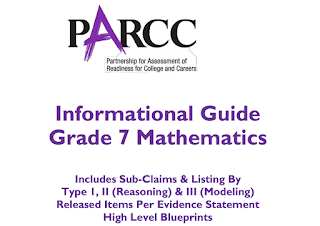## Labels

### Math 7 PARCC Reviewer 3 (7.RP.2b)

Sub-claim:
The students solve problems involving the Major Content with connections to the Standards for Mathematical Practice
Type of items: Type 1
Color Code: Peach
Evidence Statement Key: 7.RP.2b
Calculator: No
Point: 1 each (1:29)
Evidence Statement Text: Recognize and represent proportional relationships between quantities.
b. Identify the constant of proportionality (unit rate) in tables, graphs, equations, diagrams, and verbal descriptions of proportional relationships.
Clarifications, limits, emphases, and other information intended to ensure appropriate variety in tasks:
Tasks may or may not have a context.
Task sample equally across the listed representations (graphs, equations, diagrams, and verbal descriptions).
Tasks use only coordinates in Quadrant 1 and use only a positive constant of proportionality.
Sample Questions (taken from PARCC’s Practice Tests and Released Items):
EOY Released Test Item 6
PBA Released Test Item 1
PBA Released Test Item 3
Practice Test, Unit 1, Item 7
Practice Test, Unit 1, Item 11
2016 Released Item #7
2016 Released Item #111. EOY Released Test Item 6
Ed is a farmer who charges \$3.75 for 5 pounds of cabbage. The table shows the rates charged for cabbage by some other farmers. Determine whether the unit rate charged for cabbage by the other farmers is less than, equal to, or greater than the unit rate charged by Ed.
 Farmer Rate Unit Rate Less than Ed’s Unit Rate Unit Rate Equal to Ed’s Unit Rate Unit Rate Greater than Ed’s Unit Rate A \$0.50 for ½ pound B \$0.75 for 1 pound C \$1.75 for 2 ½ pounds D \$6.00 for 8 pounds
2. PBA Released Test Item 1
At this rate, what is the cost per song? Enter your answer on the blank.
\$ ________ per song.

3. PBA Released Test Item 3
What is the unit rate represented in this table?

4. Practice Test, Unit 1, Item 7
Which equation has a constant of proportionality equal to 4?
a. 4y = 4x
b. 4y = 12x
c. 3y = 4x
d. 3y = 12x

5. Practice Test, Unit 1, Item 11
Which relationships have the same constant of proportionality between y and x as in the equation y = 1/3 x? Select each correct answer.6. 2016 Released Item #7

7. 2016 Released Item #11
The values in the table show the relationship between times measured in seconds and distances measured in meters.
What is the unit rate, in meters per second, of the relationship shown in the table? Enter your answer on the blank. __________ meters per second